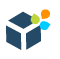DWBI.org Login / Sign Up
New Account?
Recovery
SAP Data Services

# GROUP RANK in Data Services

In this article, we will learn how to implement RANK and DENSE RANK operations on GROUP in SAP Data Services.

### Implementation

Let us take the Employee table as Source. Based on the departments, we want to RANK all the employees based on their salary. So the Employee with the highest salary of a department will get first rank and the employee with lowest salary will get the last rank. Now in the event when many employees have the same salary within a department, then they basically share the same rank. But in case of dense ranking the immediate next record will get the next consecutive rank. There will be no gap in RANKS generated. And for normal ranking the immediate next record will not get the next consecutive rank. So eventually there will be gaps in RANKS generated.

We will develop two Custom Functions that will help us to achieve the above scenario. The Job and the dataflow in both the case looks exactly identical. One dataflow will call the CF_RANK_ON_GROUP and the other will call CF_DENSE_RANK_ON_GROUP custom functions from Query transform. So let us take a look into the dataflow design first which will be pretty straight forward, followed by the custom function definitions.

1. Create a Batch Job and add a Data Flow in it.

2. Within the dataflow we take the EMP table from the Local Object Library as Source. The dataflow design for both the jobs are exactly the same.

3. Next we place a Query transform to sort the employee data based on department in ascending order and within each department we sort the employee salary in descending order.

4. Next we place a Query transform to set the Parameter values that will passed as arguments to the Custom Function to get the Ranking. So within this transform we create two additional Output Ports- one to capture the previous row value of department and the other for previous row value of salary.

``````PREV_SAL = previous_row_value( QRY_SORT.SAL )

PREV_DEPTNO = previous_row_value( QRY_SORT.DEPTNO )``````

5. Next we place a Query transform to call the Custom Function with the input parameters that we have already designed in the previous transform. Call the Function with the Input Parameters as below. This function will eventually return the Rank for each record based on the Department and salary.

``````\$PREV_SAL = QRY_SET_PARAM.PREV_SAL

\$CURR_SAL = QRY_SET_PARAM.SAL

\$PREV_DEPTNO = QRY_SET_PARAM.PREV_DEPTNO

\$CURR_DEPTNO = QRY_SET_PARAM.DEPTNO``````

Select the Return column as the Output Parameter.

6. Finally we place a Template Table as Target in the Target Datastore.

7. Now let us create two New Custom Functions from the Local Object Library to achieve the functionality of rank and dense rank on groups.

#### DENSE RANK ON GROUP

Within the Custom Function Smart Editor, we define the Parameters and Variables as below:

 Param/Variable Name Datatype Type Parameter Return int Output Parameter \$PREV_SAL decimal(7,2) Input Parameter \$CURR_SAL decimal(7,2) Input Parameter \$PREV_DEPTNO int Input Parameter \$CURR_DEPTNO int Input Variable \$HOLD_RANK int

Next we define the custom function as below and Validate the same.

``````if( \$PREV_DEPTNO IS NULL )
begin
\$HOLD_RANK = 1;
Return \$HOLD_RANK;
end

else if( \$PREV_DEPTNO = \$CURR_DEPTNO )
begin
if( \$PREV_SAL = \$CURR_SAL )
Return \$HOLD_RANK;

else
begin
\$HOLD_RANK = \$HOLD_RANK + 1;
Return \$HOLD_RANK;
end
end

else
begin
\$HOLD_RANK = 1;
Return \$HOLD_RANK;
end``````

#### RANK ON GROUP

Within the Custom Function Smart Editor, we define the Parameters and Variables as below:

 Param/Variable Name Datatype Type Parameter Return int Output Parameter \$PREV_SAL decimal(7,2) Input Parameter \$CURR_SAL decimal(7,2) Input Parameter \$PREV_DEPTNO int Input Parameter \$CURR_DEPTNO int Input Variable \$FINAL_RANK int Variable \$HOLD_RANK int

Next we define the custom function as below and Validate the same.

``````if( \$PREV_DEPTNO IS NULL )
begin
\$HOLD_RANK = 1;
\$FINAL_RANK = 1;
Return \$HOLD_RANK;
end

else if( \$PREV_DEPTNO = \$CURR_DEPTNO )
begin
if( \$PREV_SAL = \$CURR_SAL )
begin
\$FINAL_RANK = \$FINAL_RANK + 1;
Return \$HOLD_RANK;
end

else
begin
\$FINAL_RANK = \$FINAL_RANK + 1;
\$HOLD_RANK = \$FINAL_RANK;
Return \$HOLD_RANK;
end
end

else
begin
\$HOLD_RANK = 1;
\$FINAL_RANK = 1;
Return \$HOLD_RANK;
end``````